Trignometric ratios of Specific Angles - Evaluating

Chapter 8 Class 10 Introduction to Trignometry
Concept wise

What is value of sin 30?

and sin 0?

How do we remember them?

Let's learn how. We will discuss what are different values of sin, cos, tan, cosec, sec, cot at 0, 30, 45, 60 and 90 degrees and how to memorise them.

So, we have to fill this table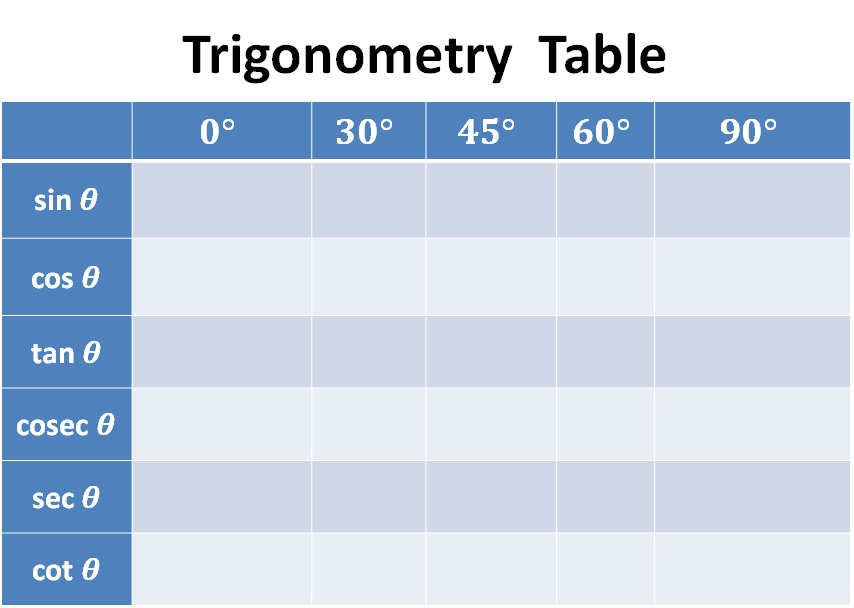## How to find the values?

To learn the table, we should first know how sin cos tan are related

We know that

• tan θ = sin θ/cosθ
• sec θ = 1/cos θ
• cosec θ = 1/sin θ
• cot θ = 1/cot θ

Now let us discuss different values

### For sin

For memorising sin 0°, sin 30°, sin 45°, sin 60° and sin 90°

We should learn it like

1. sin 0° = 0
2. sin 30° = 1/2
3. sin 45° = 1/√2
4. sin 60° = √3/2
5. sin 90° = 1

So, our pattern will be like

0, 1/2,  1/√2,  √3/2,  1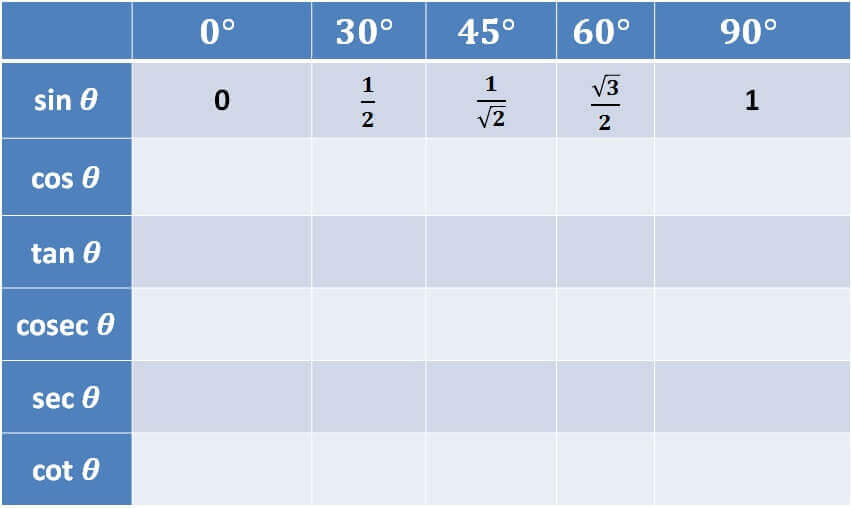### For cos

For memorising cos 0°, cos 30°, cos 45°, cos 60° and cos 90°

Cos is the opposite of sin.

We should learn it like

1. cos 0° = sin 90° = 1
2. cos 30° = sin 60° = √3/2
3. cos 45° = sin 45° = 1/√2
4. cos 60° = sin 30° = 1/2
5. cos 90° = sin 0° = 0

So, for cos, it will be like

1, √3/2,  1/√2,  1/2,  0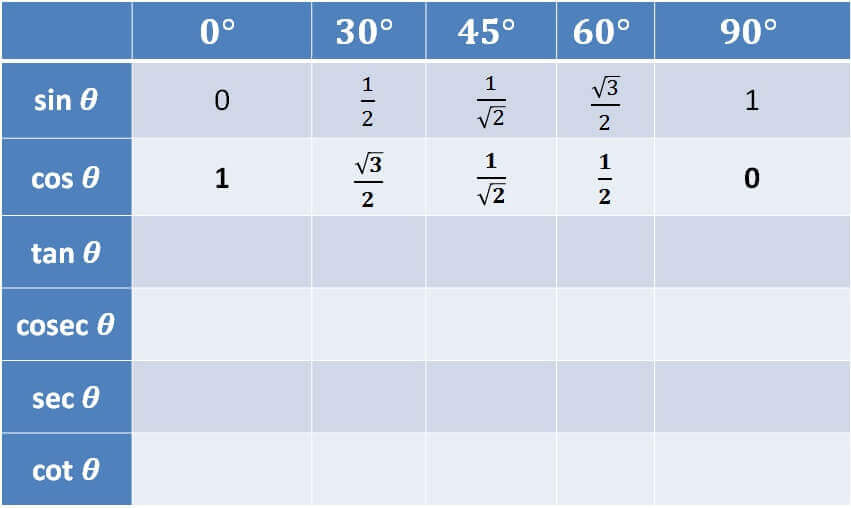### For tan

We know that tan θ = sin θ /cos θ

So, it will be

• tan 0° = sin 0° / cos 0° = 0/1 = 0
• tan 30° = sin 30° / cos 30° = (1/2)/ (√3/2) = 1/√3
• tan 45° = sin 45° / cos 45° = (1/√2)/ (1/√2) =  1
• tan 60° = sin 60° / cos 60° = (√3/2) / (1/2) =  √3
• tan 90° = sin 90° / cos 90° = 1/0 = Not Defined =

So, for tan, it is

0, 1/√3, 1, √3, ∞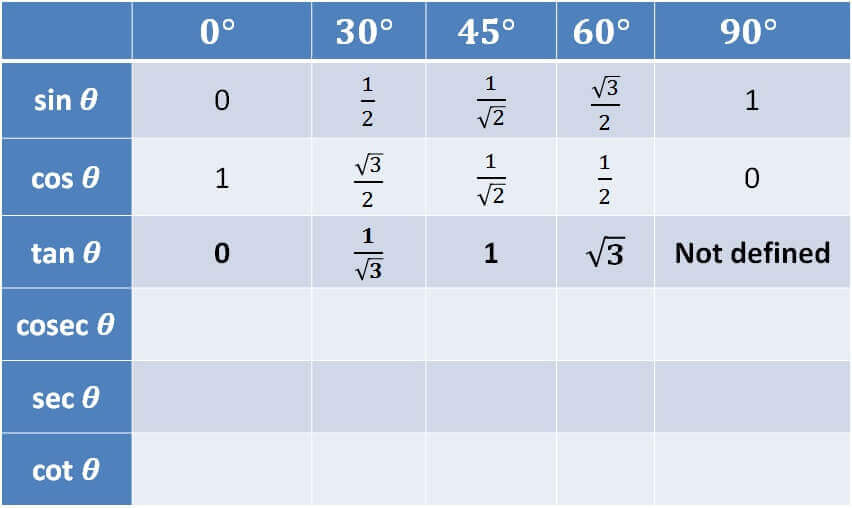### For cosec

We know that

cosec θ = 1/sin θ

For sin, we know

0, 1/2,  1/√2,  √3/2,  1

So, for cosec it will be

• cosec 0° = 1 / sin 0° = 1/0 = Not Defined =
• cosec 30° = 1 / sin 40° = 1/(1/2) =  2
• cosec 45° = 1 / sin 45° = 1/(1/√2) =  √2
• cosec 60° = 1 / sin 60° = 1/(√3/2) = 2/√3
• cosec 90° = 1 / sin 90° = 1/1 = 1

So, for cosec, it is

∞, 2, √2, 2/√3, 1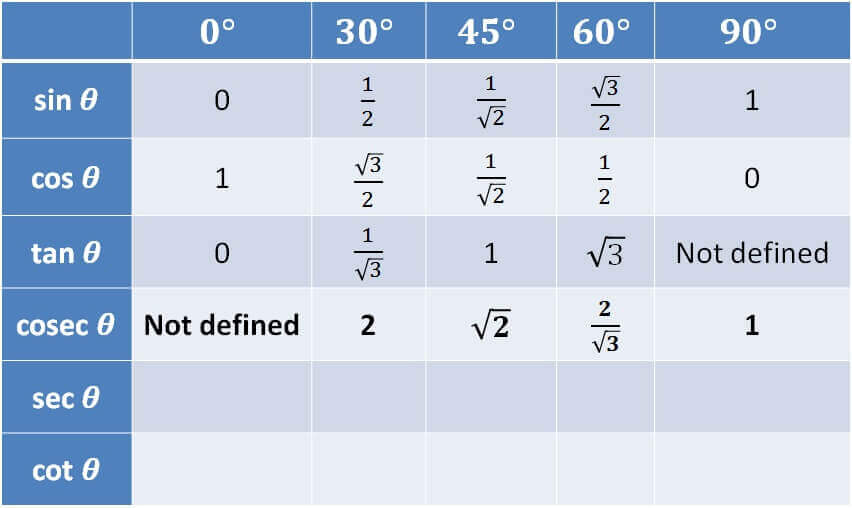### For sec

We know that

sec θ = 1/cos θ

For cos, we know

1, √3/2,  1/√2,  1/2,  0

So, for sec it will be

• sec 0° = 1 / cos 0° = 1/1 = 1
• sec 30° = 1 / cos 40° = 1/(√3/2) =  2/√3
• sec 45° = 1 / cos 45° = 1/(1/√2) =  √2
• sec 60° = 1 / cos 60° = 1/(1/2) =  2
• sec 90° = 1 / cos 90° = 1/0 = Not Defined =

So, for sec, it is

1, 2/√3, √2, 2,  ∞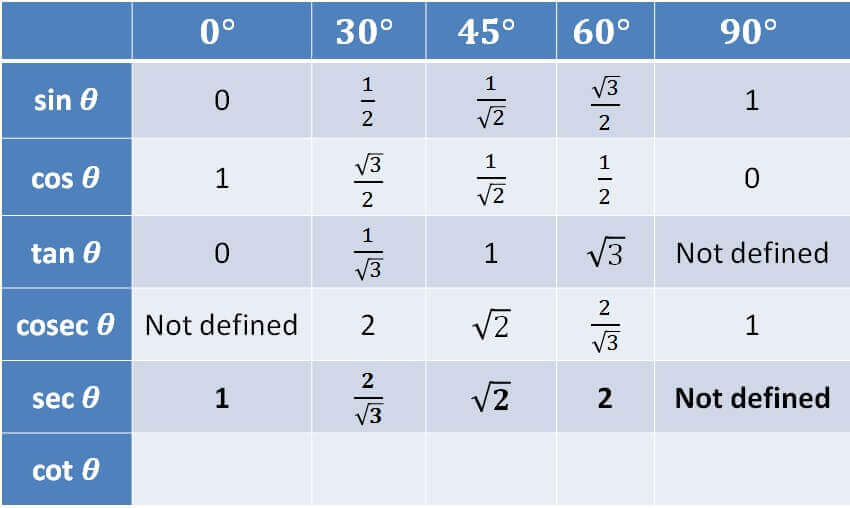### For cot

We know that

cot θ = 1/tan θ

For tan, we know that

0, 1/√3, 1, √3, ∞

So, for cot it will be

• cot 0° = 1 / tan 0° = 1/0 = Not Defined =
• cot 30° = 1 / tan 30° = 1/(1/√3) =  √3
• cot 45° = 1 / tan 45° = 1/1 = 1
• cot 60° = 1 / tan 60° = 1/√3
• cot 90° = 1 / tan 90° = 1/∞ =

So, for cot, it is

∞, √3, 1, 1/√3, 0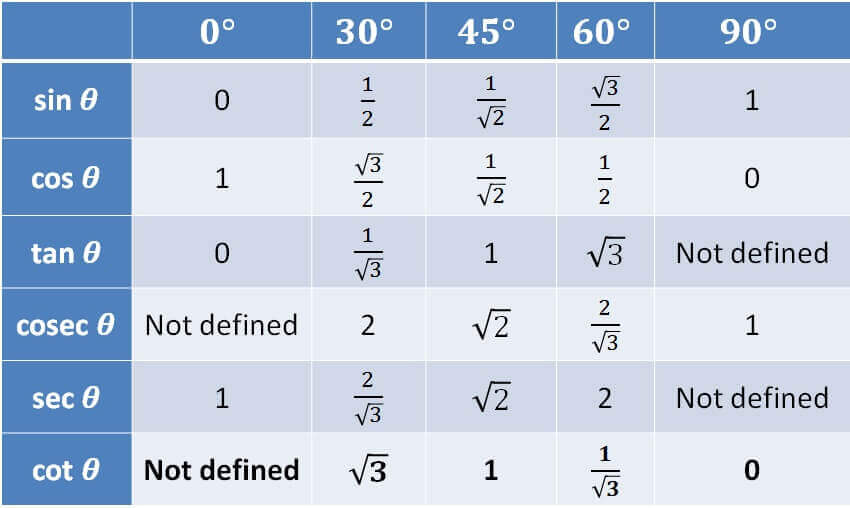So, our full table looks like this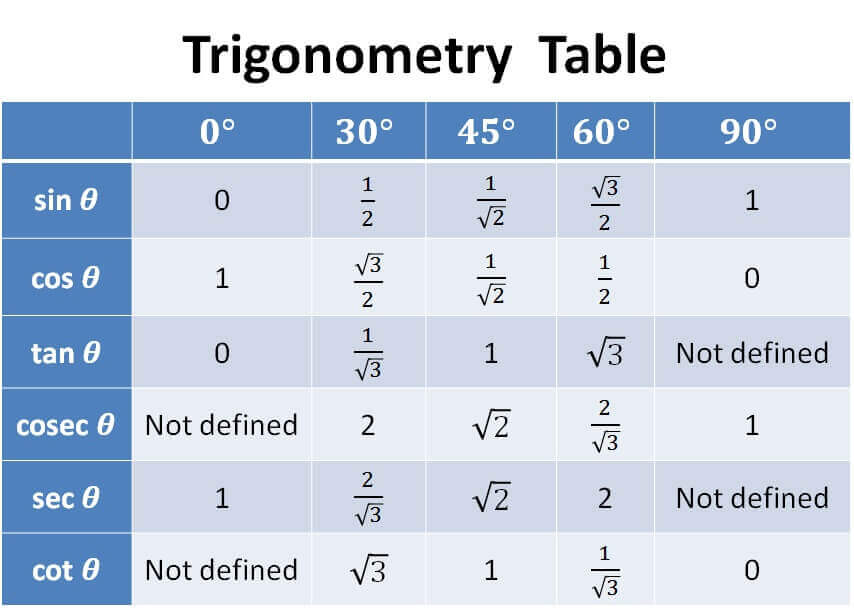You can also practice questions by clicking Next.

## Trigonometry Table

Trigonometry Table has all the values of sin, cos, tan for all angles from 0 to 90 degree.

 Radian Degree Sine Cosine Tangent Radian Degree Sine Cosine Tangent 0.000 0 0.000 1.000 0.000 0.803 46 0.719 0.695 1.036 0.017 1 0.017 1.000 0.017 0.820 47 0.731 0.682 1.072 0.035 2 0.035 0.999 0.035 0.838 48 0.743 0.669 1.111 0.052 3 0.052 0.999 0.052 0.855 49 0.755 0.656 1.150 0.070 4 0.070 0.998 0.070 0.873 50 0.766 0.643 1.192 0.087 5 0.087 0.996 0.087 0.890 51 0.777 0.629 1.235 0.105 6 0.105 0.995 0.105 0.908 52 0.788 0.616 1.280 0.122 7 0.122 0.993 0.123 0.925 53 0.799 0.602 1.327 0.140 8 0.139 0.990 0.141 0.942 54 0.809 0.588 1.376 0.157 9 0.156 0.988 0.158 0.960 55 0.819 0.574 1.428 0.175 10 0.174 0.985 0.176 0.977 56 0.829 0.559 1.483 0.192 11 0.191 0.982 0.194 0.995 57 0.839 0.545 1.540 0.209 12 0.208 0.978 0.213 1.012 58 0.848 0.530 1.600 0.227 13 0.225 0.974 0.231 1.030 59 0.857 0.515 1.664 0.244 14 0.242 0.970 0.249 1.047 60 0.866 0.500 1.732 0.262 15 0.259 0.966 0.268 1.065 61 0.875 0.485 1.804 0.279 16 0.276 0.961 0.287 1.082 62 0.883 0.469 1.881 0.297 17 0.292 0.956 0.306 1.100 63 0.891 0.454 1.963 0.314 18 0.309 0.951 0.325 1.117 64 0.899 0.438 2.050 0.332 19 0.326 0.946 0.344 1.134 65 0.906 0.423 2.145 0.349 20 0.342 0.940 0.364 1.152 66 0.914 0.407 2.246 0.367 21 0.358 0.934 0.384 1.169 67 0.921 0.391 2.356 0.384 22 0.375 0.927 0.404 1.187 68 0.927 0.375 2.475 0.401 23 0.391 0.921 0.424 1.204 69 0.934 0.358 2.605 0.419 24 0.407 0.914 0.445 1.222 70 0.940 0.342 2.747 0.436 25 0.423 0.906 0.466 1.239 71 0.946 0.326 2.904 0.454 26 0.438 0.899 0.488 1.257 72 0.951 0.309 3.078 0.471 27 0.454 0.891 0.510 1.274 73 0.956 0.292 3.271 0.489 28 0.469 0.883 0.532 1.292 74 0.961 0.276 3.487 0.506 29 0.485 0.875 0.554 1.309 75 0.966 0.259 3.732 0.524 30 0.500 0.866 0.577 1.326 76 0.970 0.242 4.011 0.541 31 0.515 0.857 0.601 1.344 77 0.974 0.225 4.331 0.559 32 0.530 0.848 0.625 1.361 78 0.978 0.208 4.705 0.576 33 0.545 0.839 0.649 1.379 79 0.982 0.191 5.145 0.593 34 0.559 0.829 0.675 1.396 80 0.985 0.174 5.671 0.611 35 0.574 0.819 0.700 1.414 81 0.988 0.156 6.314 0.628 36 0.588 0.809 0.727 1.431 82 0.990 0.139 7.115 0.646 37 0.602 0.799 0.754 1.449 83 0.993 0.122 8.144 0.663 38 0.616 0.788 0.781 1.466 84 0.995 0.105 9.514 0.681 39 0.629 0.777 0.810 1.484 85 0.996 0.087 11.430 0.698 40 0.643 0.766 0.839 1.501 86 0.998 0.070 14.301 0.716 41 0.656 0.755 0.869 1.518 87 0.999 0.052 19.081 0.733 42 0.669 0.743 0.900 1.536 88 0.999 0.035 28.636 0.750 43 0.682 0.731 0.933 1.553 89 1.000 0.017 57.290 0.768 44 0.695 0.719 0.966 1.571 90 1.000 0.000 ∞ 0.785 45 0.707 0.707 1.000